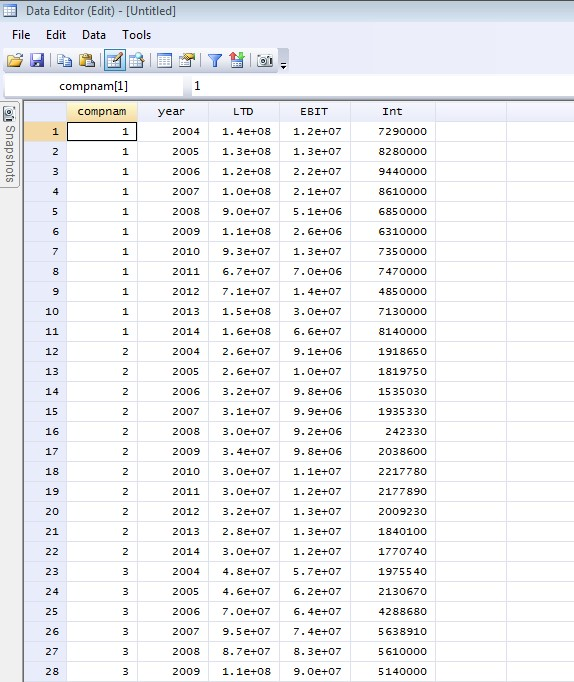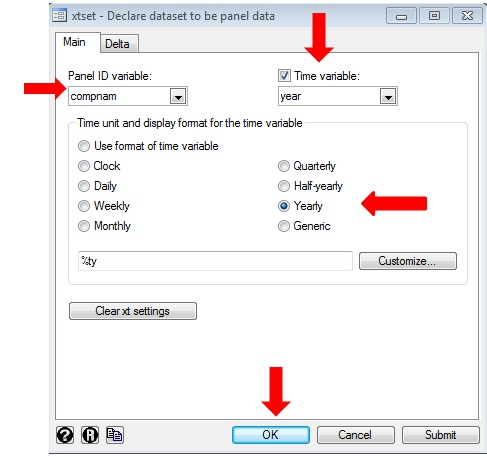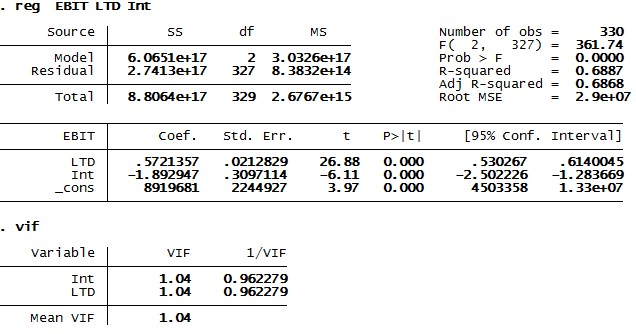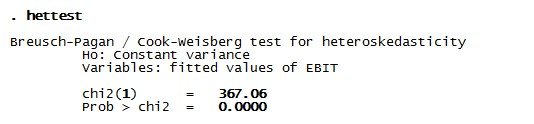# What is panel data analysis in STATA?

The previous articles in this module showed how to perform time series analysis on a dataset where observations are present for days, weeks, months, quarters or years. This article of the module explains how to perform panel data analysis using STATA. In the case of panel data, the observations are present in time and space dimensions. For instance, a survey of the same cross-sectional unit such as firm, country or state over time.

To demonstrate the idea more clearly, this article undertakes an example of 30 American firms for the period of 2004 – 2014. To start with panel data regression, take Long Term Debt (LTD), Earning Before Interest and Tax (EBIT) and Interest payments (INT) for these firms from 2004 to 2014. To start with the analysis first paste the dataset in ‘Data Editor’ window of STATA.Figure 1: Panel data set in ‘Data Editor’ window of STATA

As the figure above shows, year, LTD, EBIT and INT are in numeric form but ‘company’ is in alphabetic form and thus appearing in red color. Since this variable is now the string variable, transform it into numeric one using the following command.

`egen compnam = group(company)`

After performing the command, the ‘Data Editor’ window will transform the company name variable (company) to a numeric variable (compnam).Figure 2: Panel dataset in ‘Data Editor’ window of STATA

To start with panel data analysis, first, confirm the basic assumptions of regression analysis. Therefore check the dataset for normality, heteroscedasticity, autocorrelation, multicollinearity and unit root.

## Describe data to panel data set

Similar to time series analysis, the first step in panel data regression is to declare the dataset to panel data. In order to do so, use the below command.

`xtset compnam year, yearly`

Or follow the below steps (figure below).

1. Click on ‘Statistics’ in the main window.
2. Go to ‘Longitudinal/ panel data’.
3. Go to ‘Setup and utilities’.
4. Click on ‘Declare dataset to be panel data’.Figure 3: Pathway for declaring dataset to be panel data in STATA

A window will appear on STATA screen as shown in the figure below. Select the ‘compnam’ variable as panel variable and ‘year’ as time series variable. Select ‘Yearly’ as the display format and then click on ‘OK’.Figure 4: Declaring panel dataset for conducting panel data analysis in STATA

In the result window, the dataset shows as panel data. Also, the data shows a strong balance which means that all the cross sections have equal time dimensions (figure below).Figure 5: Panel data declaration for performing panel data analysis in STATA

## Multicollinearity

The next step is to check for the CLRM assumptions for basic regression. Starting with multicollinearity, it ensures that the correlation between independent variables is not high. Therefore it is not possible to estimate a linear combination out of predictors.  In order to check multicollinearity first perform the regression using the below command:

`reg EBIT LTD Int`

In the above syntax, EBIT as the dependent variable and LTD and INT are the independent variables. In order to check multicollinearity among independent variables, use the below command:

`vif`

The figure below shows the results of the above two commands. The first part comprises of regression results where LTD is the dependent variable and EBIT and INT are independent. Both the variables are excessive effects (large coefficients) and also significant with p values almost equal to zero.Figure 6: Regression and multicollinearity result for panel data analysis in STATA

On the other hand, the second part comprises of multicollinearity results where ‘VIF’ factor for both independent variables is less than 10. Therefore there is no multicollinearity.

## Heteroscedasticity result for panel data analysis

Similarly, check if the dataset is heteroscedastic by using the below command:

`hettest`

The below result will appear.Figure 7: Heteroscedasticity result for panel data analysis in STATA

As per the results, the null hypothesis suggests the presence of constant variance which means data is homoscedastic. However, the p-value is 0.000 which is significant enough to reject the null hypothesis. Therefore, the dataset has heteroskedastic variances. Since this is a problem as it directly violates one of the important CLRM assumptions, take appropriate measures. However, before doing so, check for normality.

## Normality

Normality ensures that residuals of variables have minimum variance. To check the same on this dataset, use the below command.

`swilk LTD EBIT Int`

1. Click on ‘Statistics’ on the main window.
2. Go to ‘Summaries, tables and test’
3. Go to ‘Distributional Plots and tests’
4. Click on ‘Shapiro-Wilk Normality test’.

The below results will appear. The null hypothesis of is that the dataset is normality distributed. However, in this case, the p values of all the variables are 0.000 which rejects the null hypothesis and thus confirms the problem of non-normality in data.Figure 8: Shapiro-Wilk normality test result for panel data analysis in STATA

This article presented all regression diagnostic tests for the panel dataset. Apart from the absence of multicollinearity, the data is not normal and even contains heterogeneous variances. However, these violations are not worrisome in case of panel data regression, which the successive articles will explain. Therefore the next article will explain the pooled regression analysis and checks its appropriateness in the present case.

### Saptarshi Basu Roy Choudhury

Senior Research Analyst at Project Guru
Saptarshi has done his M. Phil in International Trade and Development and Masters in Economics from Jawaharlal Nehru University, New Delhi. His academic interests include issues related to economics of climate change, regulation and contemporary trade theories. He has a keen interest in current affairs and likes to read and travel in his spare time.

#### Latest posts by Saptarshi Basu Roy Choudhury (see all)We are looking for candidates who have completed their master's degree or Ph.D. Click here to know more about our vacancies.